﻿ Maths stock image. Image of exams, exercise, calculate - 2781983

Maths.

Hand written maths calculations with teacher's corrections in red

Designers also selected these stock photos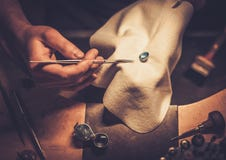Desktop for craft jewellery.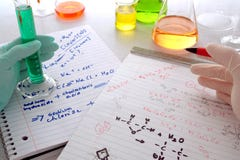Chemistry Experiment in Science Research Lab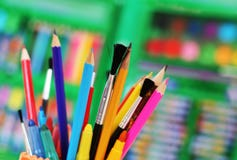Art tools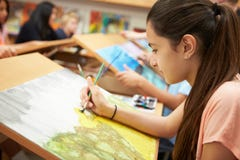Female Pupil In High School Art Class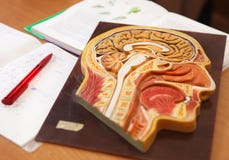Biology classroom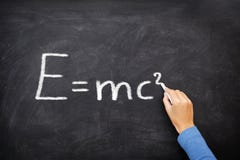Physics science formula equation blackboard, E=mcÂ²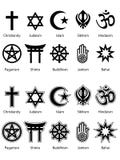Religious Symbols EPS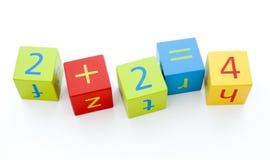Child's building blocks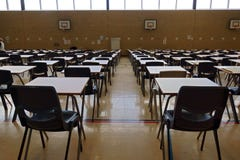Exam tables
More similar stock imagesArabic alphabet, letter za written on metallic iconZero zero one number digit maths font letter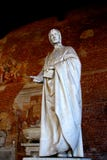PISA, ITALY - CIRCA FEBRUARY 2018: The interior of the Monumental Cemetery at the Square of MiraclesMeasurement tools and a pencil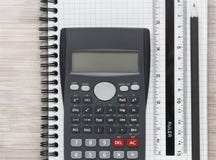Desk flat lay with calculator, ruler and pencil on a notebook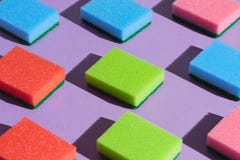Logical thinking. a pattern of many parallel sponges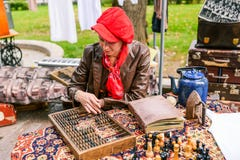Russia, city Moscow - September 6, 2014: A woman with a leather jacket and a red beret counts on a counting board. Counting board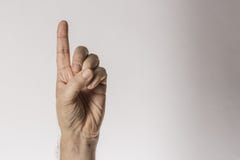Man`s hand gesture, counting number one, isolated on white background - part of set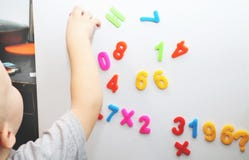A little boy is studying the magnetic numbers on the fridge. Preschooler training
More stock photos from Kmitu's portfolio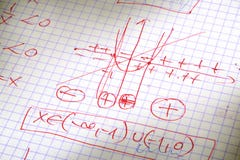Maths calculation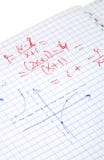Hand written maths calculations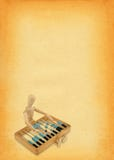Calculating the profit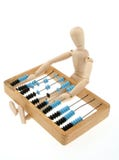Calculating the profit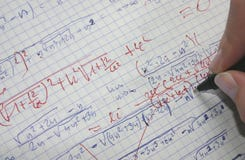Correcting maths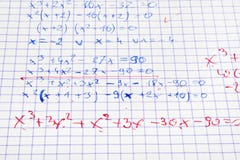Hand written maths calculations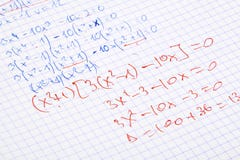Hand written maths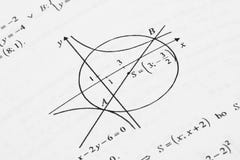Mathematics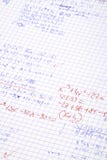Hand written maths calculations
Related categories